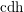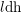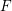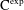Home

### Foundations (14Axx)

• Article
• ##### A BETTER COMPARISON OF$\operatorname{cdh}$ - AND$l\operatorname{dh}$ -COHOMOLOGIES
• Nagoya Mathematical Journal, Volume 236

### Local theory (14Bxx)

• Article
• ##### Ascending chain condition for$F$ -pure thresholds on a fixed strongly$F$ -regular germ
• Compositio Mathematica, Volume 155, Issue 6

### Birational geometry (14Exx)

• Article
• ##### MINIMAL MODEL THEORY FOR LOG SURFACES IN FUJIKI’S CLASS${\mathcal{C}}$
• Nagoya Mathematical Journal, First View

• Article
• ##### WAVE FRONT HOLONOMICITY OF$\text{C}^{\text{exp}}$ -CLASS DISTRIBUTIONS ON NON-ARCHIMEDEAN LOCAL FIELDS
• Forum of Mathematics, Sigma, Volume 8

### Abelian varieties and schemes (14Kxx)

• Article
• ##### ON VARIETIES WITH TRIVIAL TANGENT BUNDLE IN CHARACTERISTIC$p>0$
• Nagoya Mathematical Journal, First View

• Article
• ##### A FUNCTIONAL LOGARITHMIC FORMULA FOR THE HYPERGEOMETRIC FUNCTION$_{3}F_{2}$
• Nagoya Mathematical Journal, Volume 236

### Algebraic groups (14Lxx)

• Article
• ##### Ascending chain condition for$F$ -pure thresholds on a fixed strongly$F$ -regular germ
• Compositio Mathematica, Volume 155, Issue 6

### Projective and enumerative geometry (14Nxx)

• Article
• ##### On the$K(\unicode[STIX]{x1D70B},1)$ -problem for restrictions of complex reflection arrangements
• Compositio Mathematica, Volume 156, Issue 3

• Article
• ##### The genus-one global mirror theorem for the quintic$3$ -fold
• Compositio Mathematica, Volume 155, Issue 5

### Affine geometry (14Rxx)

• Article
• ##### ON SEPARABLE$\mathbb{A}^{2}$ AND$\mathbb{A}^{3}$ -FORMS
• Nagoya Mathematical Journal, First View